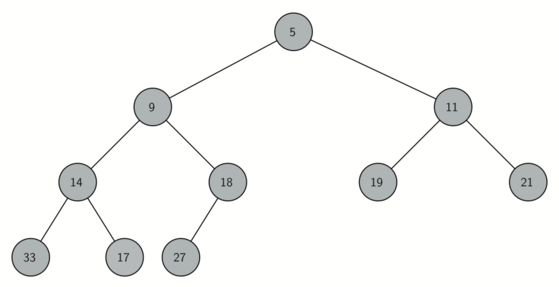# Luna Tech

Tutorials For Dummies.

# 1. Priority Queue 优先队列

Recap: Queue 的特点是 FIFO（先进先出）。

Priority Queue 是 Queue 的一个变体，和 Queue 相同的地方在于，dequeue（退出队列）的时候是从最前面开始 remove item。

# 2. Binary Heap 二叉堆

Binary Heap 看起来很像树结构，但是实际上只需要一个 list 来实现。

Binary Heap 有两个常见的变体：Min Heap（最小堆） 和 Max Heap（最大堆）。

Min Heap: 最小的在最前

Max Heap：最大的在最前

## Binary Heap 的常见功能（以 Min Heap 为例）

create, insert(k), get_min, delete, is_empty, size, heapify(list)

heapify 是把一个 list 变成 Binary Heap 的 function。

## 如何实现 Binary Heap

A balanced binary tree has roughly the same number of nodes in the left and right subtrees of the root.

$log(n)$的时间复杂度跟保持平衡有啥关系？

$log(n)$是 $log_2(n)$ 的简写，保持平衡意味着树的高度是 item 数量的一半（x/2），也就实现了 enqueue 和 dequeue 这两个操作的 $log(n)$ 时间复杂度（排序时对比的次数减少了一半）。19 的 index = 5, parent node index = (5-1)//2 = 2, parent node = 11。

9 是 parent node，index = 1，9 的左 child node index = 2+1 = 3，左 child node = 14，右 child node index = 2+2 = 4，右 child node = 18。

## Min Heap Order

• 对于 parent node 的每个 child node ，parent key ≤ child key。

## Min Heap Implementation

Insertion 逻辑

• 把新的 item 放在 list 最后，然后根据公式找到它的 parent，去跟 parent 比较大小，假如小于 parent，就和 parent 互换位置，然后继续比较，直到和 root 比完为止；

Deletion 逻辑

• 把 root 移除之后，把最后一个 item 放到 root 的位置；
• 然后新的 root 去跟 child node 进行比较，假如大于 child 就互换位置，直到位置正确为止。

Heapify 逻辑

• 假如从头开始建一个 list，把每个 item 一个个插入进去，时间复杂度是 list insertion $O(n)$ + find index O($log(n)$) = O($nlog(n)$)；
• 更好的方法是：直接建立一个完整的 list，从中间的 item 开始重新排序，_perc_down method 可以保证最大的 item 永远在 list 的后面，这个方法的时间复杂度是 O(n)；
class BinaryHeap:
def __init__(self):
self._heap = []

def insert(self, item):
self._heap.append(item)
self._perc_up(len(self._heap) - 1)

# internal method, used to make sure the order is correct by swapping parent with child when parent > child
def _perc_down(self, cur_idx):
while 2 * cur_idx + 1 < len(self._heap):
min_child_idx = self._get_min_child(cur_idx)
if self._heap[cur_idx] > self._heap[min_child_idx]:
self._heap[cur_idx], self._heap[min_child_idx] = (
self._heap[min_child_idx],
self._heap[cur_idx],
)
else:
return
cur_idx = min_child_idx

def _get_min_child(self, parent_idx):
if 2 * parent_idx + 2 > len(self._heap) - 1:
return 2 * parent_idx + 1
if self._heap[2 * parent_idx + 1] < self._heap[2 * parent_idx + 2]:
return 2 * parent_idx + 1
return 2 * parent_idx + 2

def delete(self):
self._heap, self._heap[-1] = self._heap[-1], self._heap
result = self._heap.pop()
self._perc_down(0)
return result

# use _perc_down to ensure large item is after small item
def heapify(self, not_a_heap):
self._heap = not_a_heap[:] # deep clone
cur_idx = (len(self._heap) - 1) // 2 # find middle item index
while cur_idx >= 0:
self._perc_down(cur_idx)
cur_idx = cur_idx - 1 # out of loop when len(self._heap) = 0

def is_empty(self):
return not bool(self._heap)

def __len__(self):
return len(self._heap)

def __str__(self):
return str(self._heap)

a_heap = BinaryHeap()
a_heap.heapify([9, 5, 6, 2, 3])

while not a_heap.is_empty():
print(a_heap.delete())


#### 如何证明我们可以用 O(n) 的时间复杂度来进行 heapify？

The key to understanding that you can build the heap in 𝑂(𝑛) is to remember that the log𝑛 factor is derived from the height of the tree. For most of the work in heapify, the tree is shorter than log𝑛.

Using the fact that you can build a heap from a list in 𝑂(𝑛) time, you will construct a sorting algorithm that uses a heap and sorts a list in 𝑂(𝑛log𝑛).